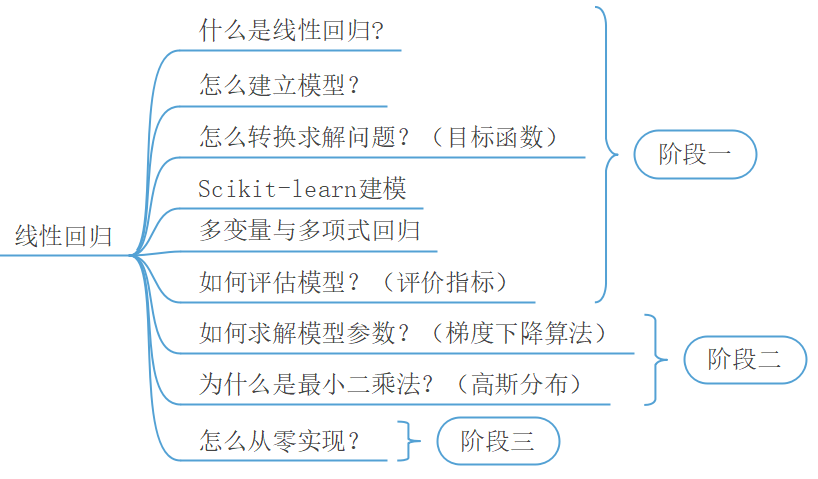### 2.1模型的建立与求解

#### 2.1.1理解线性回归模型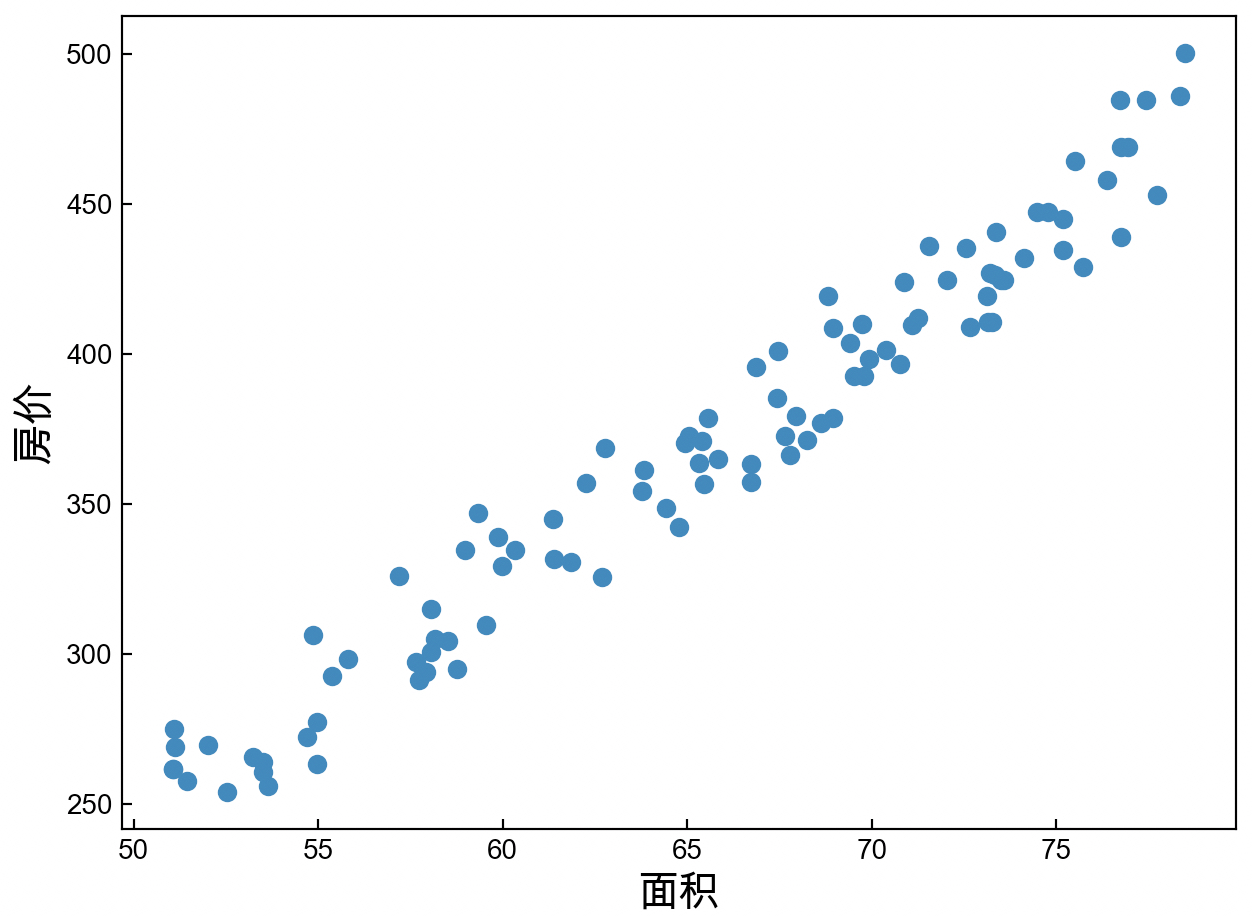#### 2.1.4 sklearn简介#### 2.1.5安装sklearn以及其它库

##### 2) 安装Python包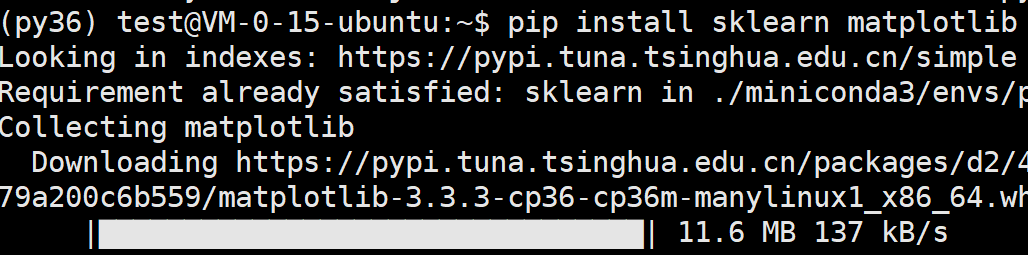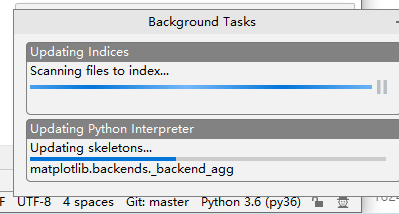### 2.1.6线性回归示例代码

##### 1) 导入包

31import numpy as np2from sklearn.linear_model import LinearRegression3import matplotlib.pyplot as plt
##### 2) 制作样本（数据集）

61def make_data():2    np.random.seed(20)3    x = np.random.rand(100) * 30 + 50  # 面积4    noise = np.random.rand(100) * 505    y = x * 8 - 127 - noise              # 价格6    return x,y

##### 3) 定义模型并求解

61def main(x, y):2    model = LinearRegression()            # 定义模型3    x = np.reshape(x, (-1, 1))4    model.fit(np.reshape(x, (-1, 1)), y)# 求解模型5    y_pre = model.predict(x) # 预测6    print(y_pre)

##### 3) 运行结果

51if __name__ == '__main__':2    x, y = make_data()3    main(x, y)      4    #参数w=[7.97699647],b=-154.318850060615555    #面积50的房价为： [244.53097351]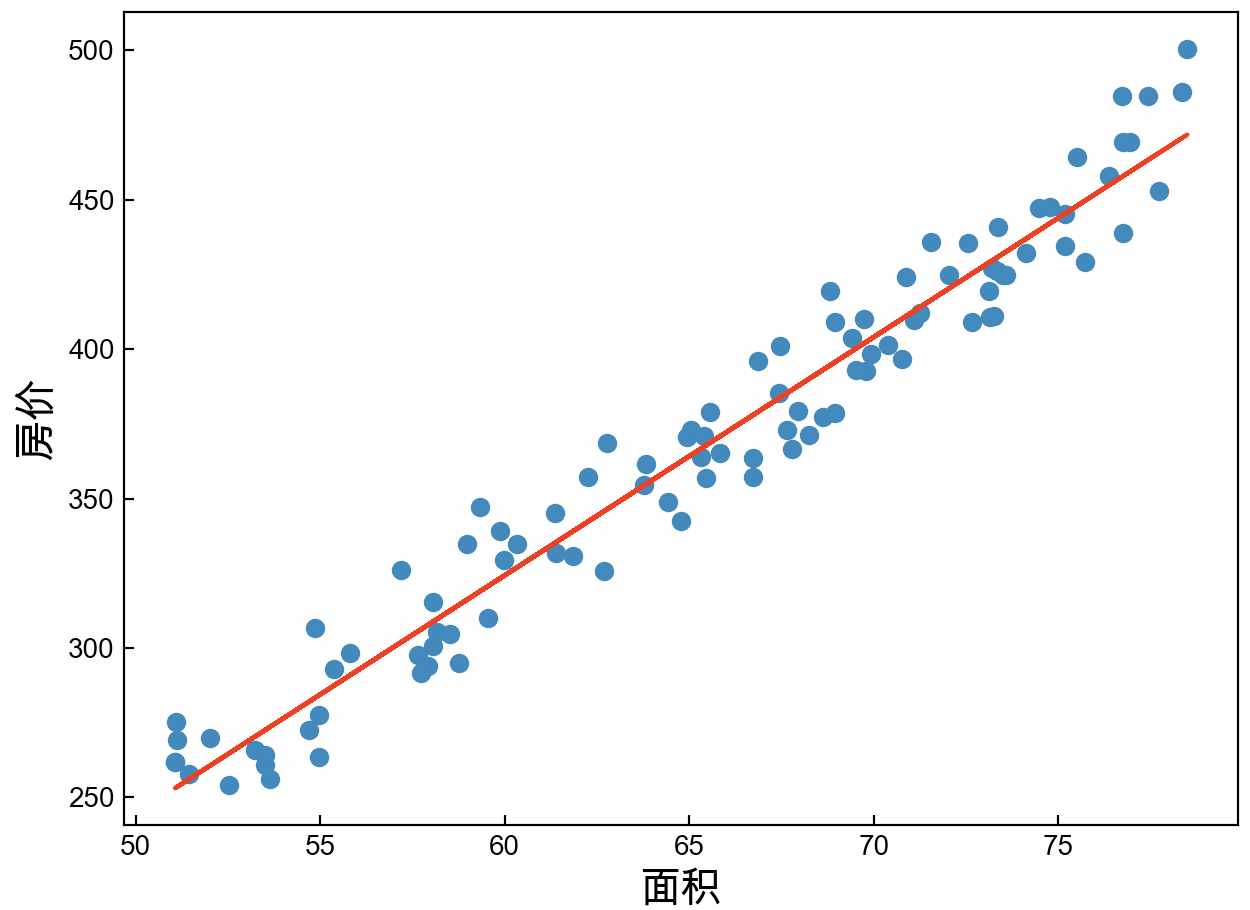### 2.2 多变量线性回归

#### 2.2.3 多变量回归示例代码

##### 1) 导入数据集

51def load_data():2    data = load_boston()3    x = data.data4    y = data.target5    return x, y
##### 2) 求解与结果

81def train(x, y):2    model = LinearRegression()3    model.fit(x,y)4    print("权重为：",model.coef_,"偏置为：",model.intercept_)5    print("第12个房屋的预测和真实价格：",model.predict(x[12,:].reshape(1,-1)))6    7#参数w=[7.97699647],b=-154.318850060615558#面积50的房价为： [244.53097351]

### 2.3 多项式回归

#### 2.3.1 理解多项式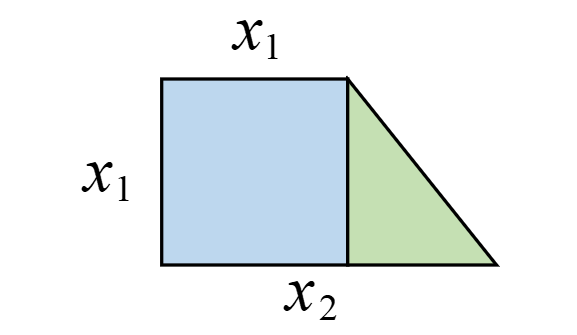#### 2.3.3 多项式回归示例代码

##### 1) 特征转化

51from sklearn.preprocessing import PolynomialFeatures2a = [[3, 4], [2, 3]]  3model = PolynomialFeatures(degree=2,include_bias=False)4b = model.fit_transform(a)5print(b)#输出结果：[[ 3.  4.  9. 12. 16.] [ 2.  3.  4.  6.  9.]]
##### 2) 构造数据集

61def make_data():2    np.random.seed(10)3    x1 = np.random.randint(5, 10, 50).reshape(50, 1)4    x2 = np.random.randint(10, 16, 50).reshape(50, 1)5    x,y = np.hstack((x1, x2)), 0.5 * (x1 + x2) * x16    return x, y

##### 3) 建模求解与结果

xxxxxxxxxx71def train(x, y):2    poly = PolynomialFeatures(degree=2, include_bias=False)3    x_mul = poly.fit_transform(x)4    model = LinearRegression()5    model.fit(x_mul, y)6    print("权重为：", model.coef_)#[[0.  0.  0.5   0.5   0]]7    print("偏置为：", model.intercept_) # [0.]

### 2.4 回归模型评估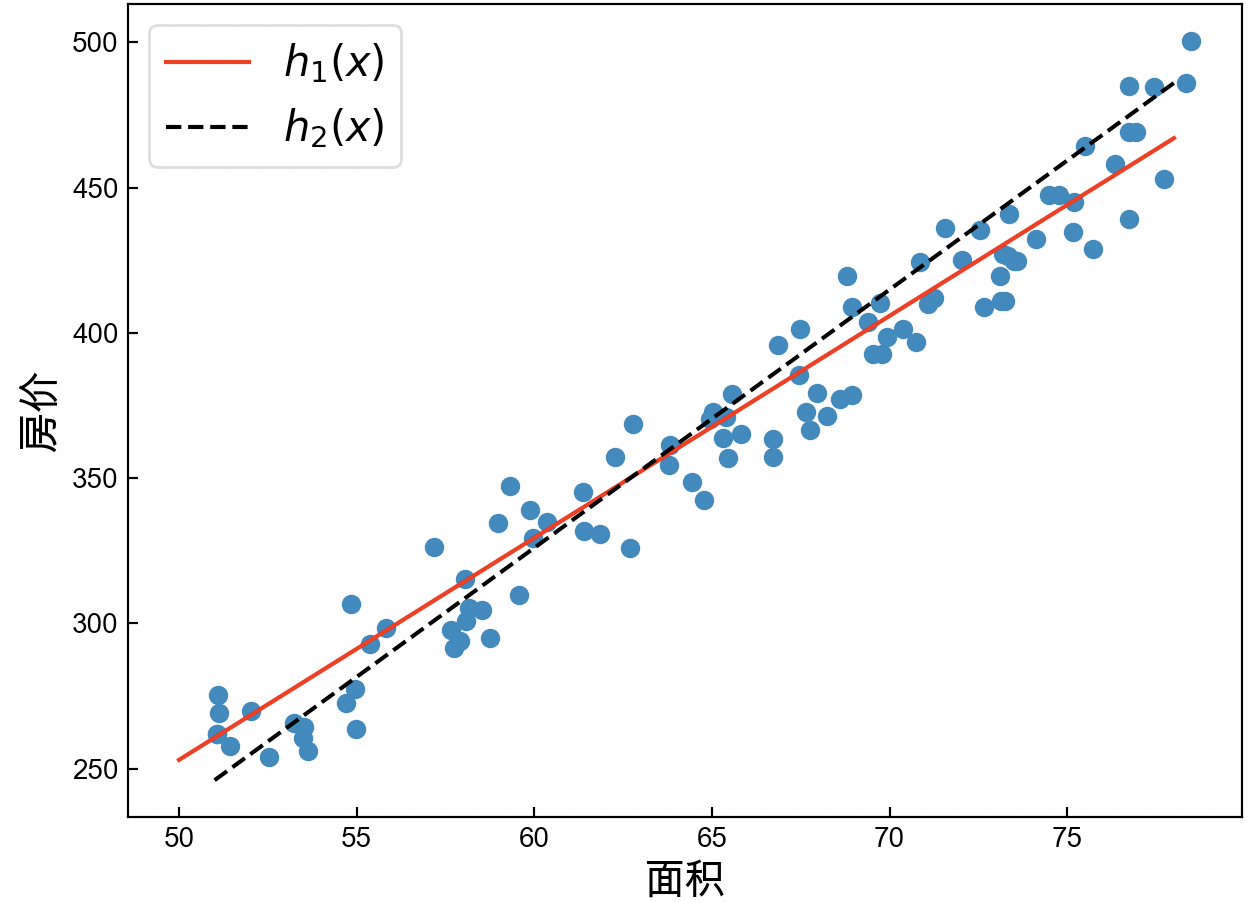#### 2.4.1常见回归评估指标

##### 1) 平均绝对误差（MAE）

MAE用来衡量预测值与真实值之间的平均绝对误差，定义如下：

xxxxxxxxxx21def MAE(y, y_pre):2    return np.mean(np.abs(y - y_pre))
##### 2) 均方误差（MSE）

MSE用来衡量预测值与真实值之间的误差平方，定义如下：

xxxxxxxxxx21def MSE(y, y_pre):2    return np.mean((y - y_pre) ** 2)
##### 3) 均方根误差（RMSE）

RMSE是在MSE的基础之上开根号而来，其定义如下：

xxxxxxxxxx21def RMSE(y, y_pre): 2    return np.sqrt(MSE(y, y_pre))
##### 4) 平均绝对百分比误差（MAPE）

MAPE和MAE类似，只是在MAE的基础上做了标准化处理，其定义如下：

xxxxxxxxxx21def MAPE(y, y_pre):2    return np.mean(np.abs((y - y_pre) / y))
##### 5) $R^2$评价指标

xxxxxxxxxx41def R2(y, y_pre):2    u = np.sum((y - y_pre) ** 2)3    v = np.sum((y - np.mean(y_pre)) ** 2)4    return 1 - (u / v)

#### 2.4.2 回归指标示例代码

xxxxxxxxxx41def train(x, y):2    model = LinearRegression()3    model.fit(x, y)4    y_pre = model.predict(x)

### 2.5梯度下降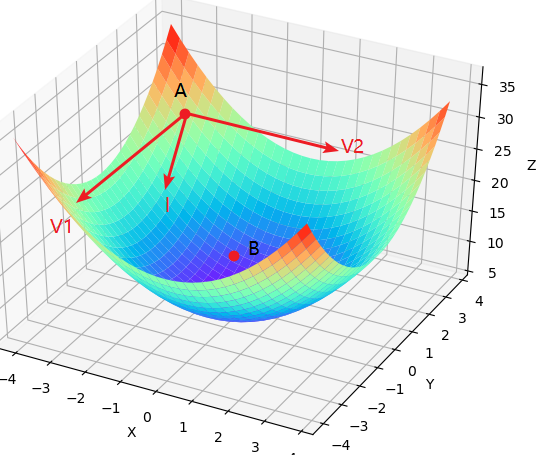### 2.5.1方向导数与梯度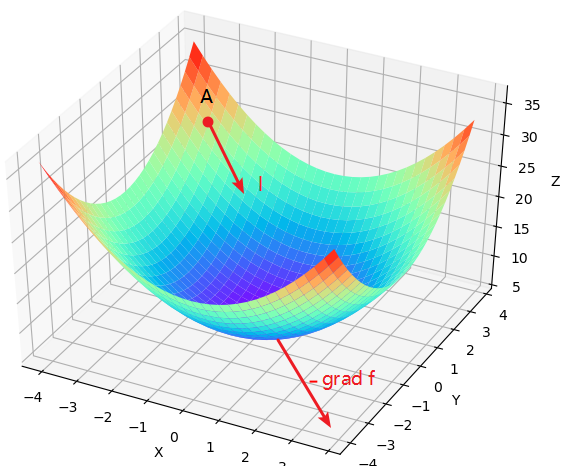### 2.5.2梯度下降算法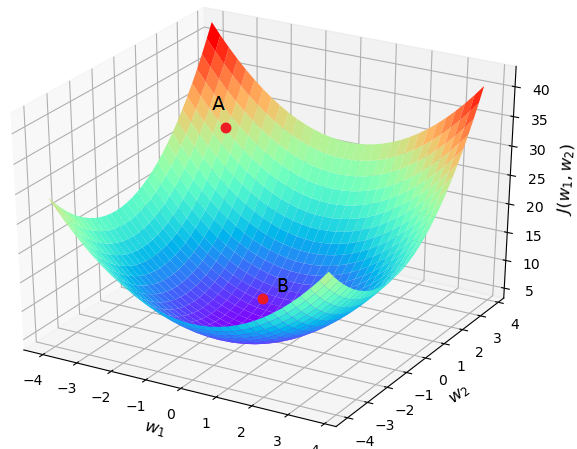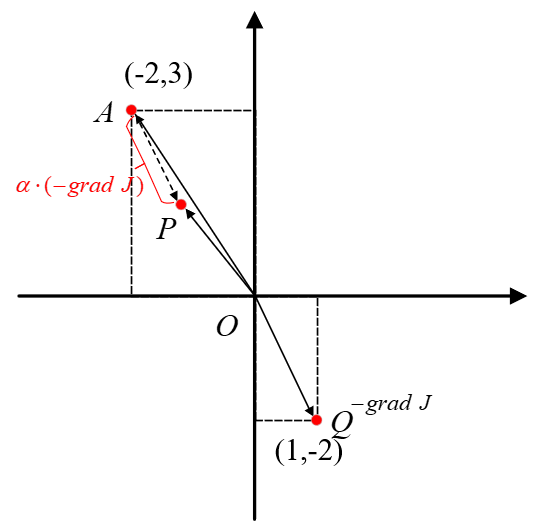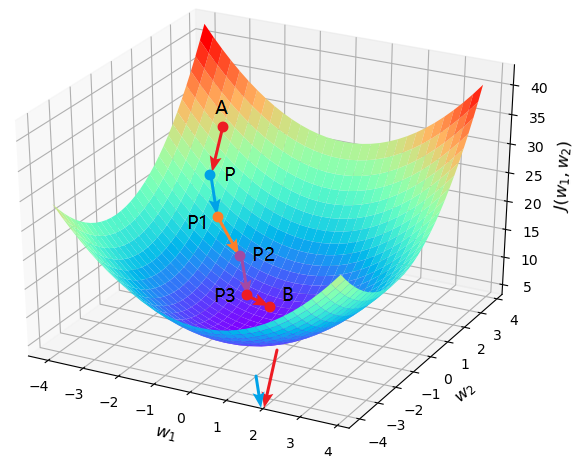​x1def cost_function(w1, w2):2    J = w1 ** 2 + w2 ** 2 + 2 * w2 + 53    return J4def compute_gradient(w1, w2):5    return [2 * w1, 2 * w2 + 2]6​7def gradient_descent():8    w1, w2 = -2, 39    jump_points = [[w1, w2]]10    costs = [cost_function(w1, w2)]11    step = 0.112    print("P:({},{})".format(w1, w2), end=' ')13    for i in range(20):14        gradients = compute_gradient(w1, w2)15        w1 = w1 - step * gradients16        w2 = w2 - step * gradients17        jump_points.append([w1, w2])18        costs.append(cost_function(w1, w2))19        print("P{}:({},{})".format(i + 1, round(w1, 3), round(w2, 3)), end=' ')20    return jump_points, costs21​22if __name__ == '__main__':23    jump_points, costs = gradient_descent()24    plot_surface_and_jump_points(jump_points, costs)25    26# 部分结果：P:(-2,3) P1:(-1.6,2.2) P2:(-1.28,1.56).....P20:(-0.023,-0.954)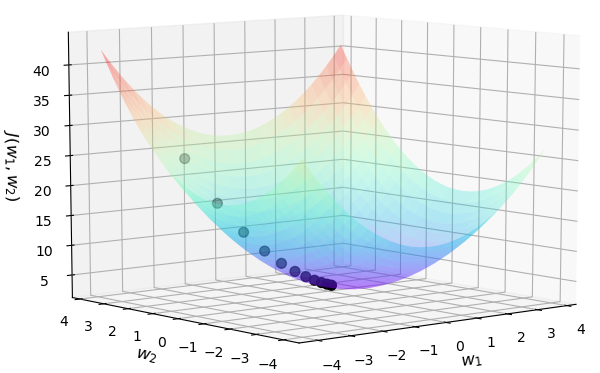### 2.6正态分布

#### 2.6.1一个问题的出现

17、18世纪是科学发展的黄金年代，微积分的发展和牛顿万有引力定律的建立，直接的推动了天文学和测地学的迅猛发展。这些天文学和测地学的问题，无不涉及到数据的多次测量、分析与计算。很多年以前，学者们就已经经验性的认为，对于有误差的测量数据，多次测量取算术平均是比较好的处理方法，并且这种做法现在我们依旧在使用。虽然当时缺乏理论上的论证，且也不断的受到一些人的质疑，但取算术平均作为一种直观的方式，被使用了千百年。 同时，算术平均在多年积累的数据处理经验中也得到相当程度的验证，被认为是一种良好的数据处理方法，但是在当时却没人能给出为什么。 1805年，勒让德提出了一种方法来解决这个问题，其基本思想认为测量中存在误差，且让所有方程的累积误差为$\sum{{{(\hat{y}-y)}^{2}}}$，其中$\hat{y}$为观测值，$y$为理论值。然后通过最小化累积误差来计算得到理论值。即设真实值为$\theta$${{x}_{1}},{{x}_{2}},...,{{x}_{n}}$分别为$n$次独立观测后的测量值，每次测量的误差为$e_{i}={x}_{i}-\theta$，按照勒让德提出的方法，累计误差为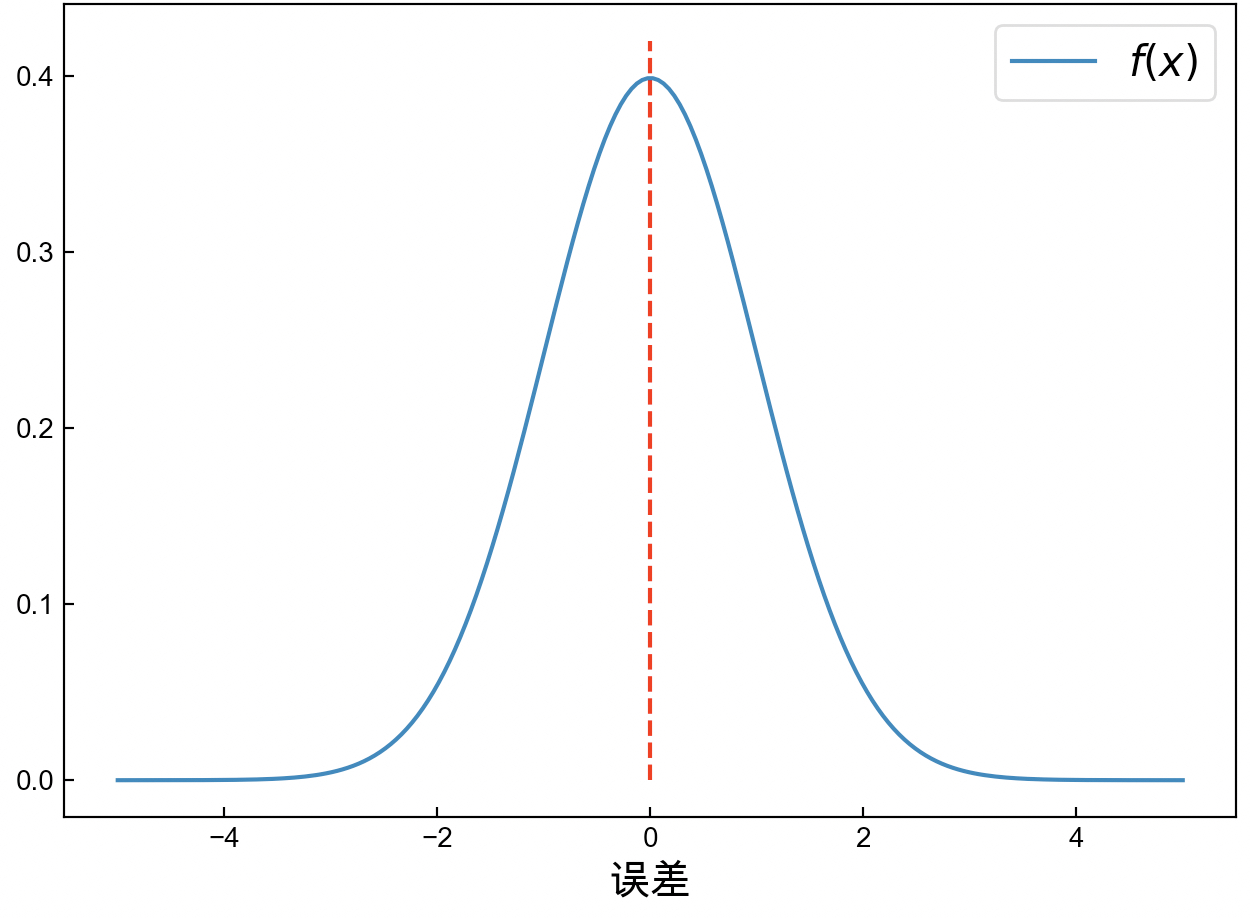#### 2.6.2 正态分布

1801年1月，天文学家朱塞普·皮亚齐发现了一颗从未见过的光度为8等的星在移动，这颗现在被称作谷神星（Ceres）的小行星在夜空中出现 6 个星期，扫过八度角后就在太阳的光芒下没了踪影无法观测。由于留下的观测数据有限难以计算出它的轨迹，所以天文学家们也因此无法确定这颗新星是彗星还是行星。不过这个问题很快成了学术界关注的焦点。高斯当时已经是很有名望的年轻数学家，这个问题引起了他的兴趣。高斯以其卓越的数学才能创立了一种崭新的行星轨道的计算方法，一个小时之内就计算出了谷神星的轨道，并预言了他在夜空中出现的时间和位置。1801年12月31日夜，德国天文爱好者奥伯斯，在高斯预言的时间里，用望远镜对准了这片天空，果然不出所料，谷神星出现了。

### 2.7 目标函数推导

#### 2.7.2 求解梯度

$y^{(i)}$表示第$i$个样本的真实值；$\hat{y}^{(i)}$表示第$i$个样本的预测值；$W$表示权重（列）向量，$W_j$表示其中一个分量；$X$表示数据集，形状为$m\times n$$m$为样本个数，$n$为特征维度；$x^{(i)}$为一个（列）向量，表示第$i$个样本，